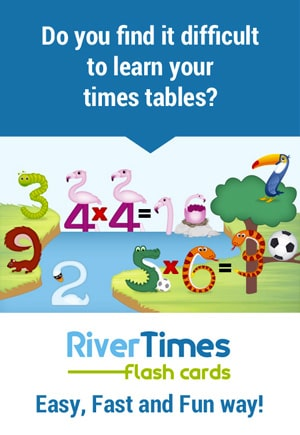# Printable Green multiplication Chart (1-10) - Free

1 Review

Here is the Printable Multiplication Chart (PDF) from the 1 time table up to the 10 times table, it's a Free resource. Its pretty green color makes it more attractive than a classic chart in black and white. You can use it as a reminder or to learn your times tables up to 10x10 multiplication. Feel free to color it to memorize more easily.

Discover our Wide range of Printable Multiplication Charts as well as our Tricks to help you learn your tables.

A Complete multiplication Chart, like this one, is a great way to learn the tables. When you think you know them, we advise you to practice with a Blank multiplication Chart to fill in, it is an effective way to finally know by heart your multiplication tables.

Click on the button below to print for Free the Green multiplication Chart (1-10) in Pdf format.

Print the Green
Chart 1-10 (PDF)

## Green Multiplication Tables Chart (1-10) in PDF format

This multiplication chart is a 10x10 grid with rows of numbers colored bright green and the other cells are light green. It is in standard A4 size so that you can easily print it at home. By clicking on the button above, you can print or download this PDF Chart. Then post it on your bedroom wall or put it into your school bag. You can also glue it on a cardboard support (and eventually laminate it) so that it lasts longer!

This Chart is a grid containing the multiplication tables from 1 to 10, it is also called « Pythagorean table ». The Pythagorean table is a visual representation of the multiplication tables that is very useful to quickly find the result of a multiplication.

### How to read this Chart ?

To find the result of a multiplication, simply look for the intersection between the horizontal line corresponding to the first multiplication number and the vertical line corresponding to the 2nd number. You can dragg your finger along the line to the intersection if that helps.
Find our illustrated & detailed explanation here: How to read a Multiplication table Chart ?.

## Our Tricks for learning your multiplication Tables from 1 up to 10

### No need to learn the whole multiplication Chart

There are many duplicates in a chart. Indeed, in a multiplication operation, you can invert the numbers while obtaining the same result.
For example: 7 x 6 = 6 x 7 = 42
So if you know the result of 6 x 7, you also know the result of 7 x 6!

There is therefore no need to learn duplicates. It is also not necessary to learn by heart the 1 Time and 10 Times tables because they are very easy! Once all duplicates have been removed (as well as the 1 Time and 10 Times tables) there are only 36 multiplications left to know (instead of 100)!

### Bring colour into your Chart!

To make the learning of the multiplication tables easier for you, the ideal is to colour the chart by assigning a different colour to each table. To save time, print a colored chart as our Multiplication Chart Color-coded or choose one of our Colorful multiplication Charts (1-10).

To create a personalized chart with your favorite colors, print our Black and White Multiplication Chart 10x10 and let's get out your felt pens! Follow our coloring tips below:
For example: If you assign the green to the number 3, you will have to color in green the following cells: 3x3, 3x4, 3x5, 3x6, 3x7, 3x8, 3x9 (horizontal line) as well as 4x3, 5x3, 6x3, 7x3, 8x3, 9x3 (vertical line). 3x2, belongs to the 2 times table, so color it using the color of the 2 times table. Indeed, the result of 3x2 is equal to the result of 2x3, it is therefore useless to learn both, when you know the first, you find the second.
Note: There is no need to color the cells corresponding to the 1 and 10 times table because the results are very easy and you will not need to learn them by heart!

### Tricks for the tables from 1 to 10

#### 1 Time table

When a number is multiplied by 1, the result is always the number (Example: 8 x 1 = 8).

#### 2 Times & 4 Times table

The common point of these 2 tables is that the result always ends with 0,2,4,6 or 8.

#### 5 Times table

The result of this table always ends with 0 or 5.

#### 6 Times table

It's the table that rhymes! Indeed, in this table, there are 3 multiplications which rhyme and which will be thus much easier to memorize than the others. They are 6x4=24, 6x6=36, 6x8=48. The ideal is to repeat aloud several times these 3 multiplications and you will see that, thanks to the rhymes, you will learn them very fast!

#### 9 Times table

When you multiply a number by 9, the result always starts with the number from which you have subtracted 1 (number - 1). Example: 9 x 8 = 72 (because 8-1 =7). Moreover, the sum of the 2 digits of the result is always equal to 9. Example: 9 x 8 = 72 (7+2=9).

#### 10 Times table

When a number is multiplied by 10, the result is the number followed by a zero (Example: 8 x 10 = 80).